# MATLAB Colon## Introduction to MATLAB Colon

‘Colon’ is used as an operator in MATLAB programming and is one of the frequently used operators. This operator comes into the picture in order to create vectors defining with simple expressions, specifying‘for’ iterations or subscribing arrays, or getting access to a set of elements of an existing vector in a sequence. When a vector is created to index into a cell or structure type array using the colon operator in the form of ‘cellName{:}’ or ‘structName(:).fieldName)’, MATLAB results in multiple outputs putting in a comma-separated list.

### Syntax

Various forms of syntax are supported to use the operator ‘:’. The functionalities of different syntaxes are described as below:

 Syntax Description li = j:k This syntax is used to create aunit spaced vector list i.e. values with increment value ‘1’, consisting of the elements as [j,j+1,…,k]. li= j:i:k This syntax is used to create a regularly-spaced vector list ‘li’ using values with increment value ‘i’, consisting of the elements [j,j+1,…,k]. M(:,n) This syntax is used to store the nth column of matrix M. M(m,:) This syntax is used to store the mthrow of matrix M. M(:) This syntax can be used to reshape the element ‘M’ into a vector containing a single column. M(j:k) This syntax can be used to apply the vector list having the elementsj: kin order to index into matrix M. This is equivalent to forming vector as [M(j), M(j+1), …, M(k)]. M(:,:,p) This syntax can be used to store/extract the data from a three-dimensional array A set in the pth page. M(:,:) This syntax can be used to reshapethe elements of matrix ‘M’ into a matrix of two-dimensional. M(:,j:k) This syntax can be used to include the subscripts present in the first dimension and to use the vector having elements j:k, for indexing the second dimension. This results in a matrix having columns as [M(:,j), M(:,j+1), …, M(:,k)].

### Examples to Implement MATLAB Colon

Below are some examples mentioned:

#### Example #1

Code:

`list_name = -3:3`

Output: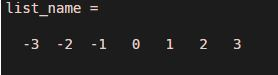Explanation: The command has generated a list of values from -3 to 3 having different between 2 consecutive elements as ‘1’.

#### Example #2

Code:

`list_name = -3:3:30`

Output: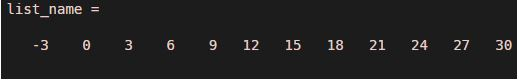Explanation: The command has generated list of values from -3 to 30 having different between 2 consecutive elements as ‘3’.

#### Example #3

Code:

`Mat_A = magic(3)Mat_A(2,:)`

Output: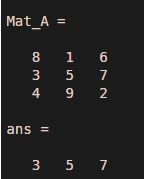Explanation: The command has displayed the elements from the second row of the matrix ‘Mat_A’

#### Example #4

Code:

`Mat_A = magic(3)Mat_A(:,2)`

Output: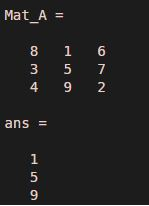Explanation: The command has displayed the elements from the second column of the matrix ‘Mat_A’

#### Example #5

Code:

`Mat_A = magic(3)Mat_A(:)`

Output: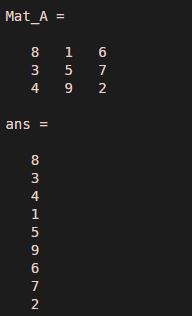Explanation: The command has displayed the elements of the matrix ‘Mat_A’ in a single column.

#### Example #6

Code:

`Mat_A = magic(4)Mat_A(2:4)`

Output: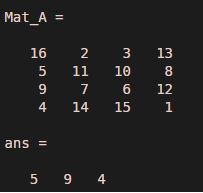Explanation: The command has displayed the elements of the matrix ‘Mat_A’ indexed between 2 to 4.

### Application of MATLAB Colon

Below are the applications:

#### 1. Using colon to create a list

A vector with evenly-spaced numbers can be generated using a colon operator.

Code:

`Mat_A =1:2:10`

Output: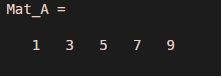#### 2. Creating a vector with only column format

The colon operator can be used to transform the input of the row vector type to that of the column vector type. The colon operator can also be used with reshaping or permute functions to create a column vector.

Code:

```M = rand(3,2,6,4); M32sum = sum(M(3,2,:)) M32sumAll = sum(M(3,2,:,:)) %Creating column vector using reshape method M32vecR = reshape(M32sumAll,[],1) %Creating column vector using %permute method M32vecP = permute(M32sumAll, [4 1:3]) %Creating column vector using only colon operator M32vec = M32sumAll(:) allthesame = isequal(M32vec, M32vecP, M32vecR)```

Output: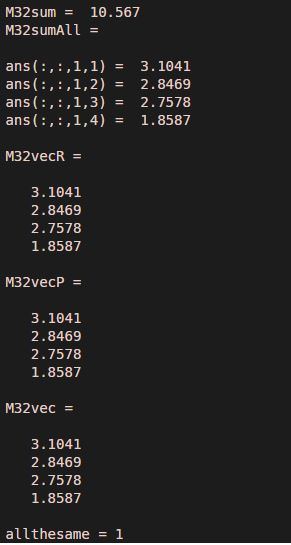#### 3. Maintaining the shape of an array during assignment operation

The application of the assignment operator in the assignment operation on the input matrix, assures the shape of the array remains unchanged.

Code:

`M32sumAll(:) = [1 2; 3 5]`

Output: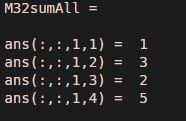Note: In order to maintain the shape of the input array, the number of elements being assigning to the input array should be the same as the number of elements in the existing array input. The values from the right-hand side get assigned to the input array in the left-hand side in the form of a column vector. A scalar value can also be used as the right-hand side operand in the assignment operation. In that case, MATLABapplies a scalar expansion for the left-hand side to be filled.

#### 4. Working with all the entries in specified dimensions

The colon operator can also be used to manipulate specific dimensions of the input array.

Code:

`M = zeros(3,3,3)M(:) = 1:numel(M)M1 = M(:,[2:size(M,2) 1],:)`

Output: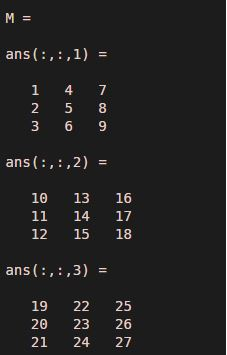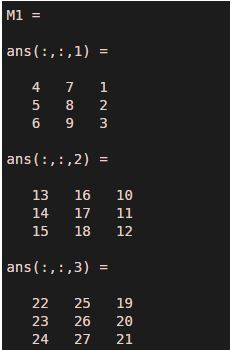Explanation: The indices of the elements in the input matrix ‘M’ is redefined and created new input matrix M1 with the application of the colon operator.

#### 5. Create Unit-Spaced Vector

Using a colon operator, a vector having a list of consecutive numbers within a specified range, can be generated using the syntax li=j: k.

Code:

`list_vector = 1:15`

Output: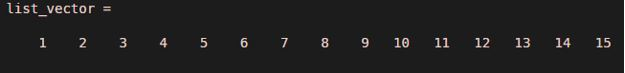Explanation: A list of having a number from 1 to 15 is generated from the MATLAB command using a colon operator.

#### 6. Create a Vector with Specified Increment

Using colon operator, a vector having a list of numbers having an equal difference between two consecutive numbers within a specified range can be generated using the syntax li=j:i: k.

Code:

`list_vector = 1:3:15`

Output: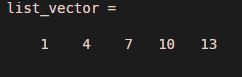Explanation: A list of having numbers from 1 to 15 with a common difference of 3, are generated from the MATLAB command using a colon operator.

#### 7. Specify for-loop Iterations

Colon operator is also used in designing the looping operation in MATLAB programming.

Code:

```for m = 1:4 m^3+3 end```

Output: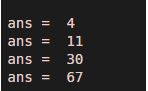In case I being a non-integer and k is not equals to j+m*i, in the command form of li=j:i:k, floating-point arithmetic determines about colon including the endpoint k or not.

If no scalar array is specified, then MATLAB assumes i:k as j(1): i(1):k(1).

li = colon(j,k) and li = colon(j,i,k) are rarely used alternate ways for the commands li=j:k and li=j:i:k respectively.While implementing class, these syntaxes can be used to apply operator overloading.

Linspaceexhibit similar behavior to that of colon operator. The difference lies aslinespacegives direct control over the number of points and endpoints are always gets included.

Logspace, the sibling function is used to generate values being logarithmically spaced.

### Recommended Articles

This is a guide to MATLAB Colon. Here we discuss an introduction to MATLAB Colon, syntax, examples and application. You can also go through our other related articles to learn more –

The post MATLAB Colon appeared first on EDUCBA.